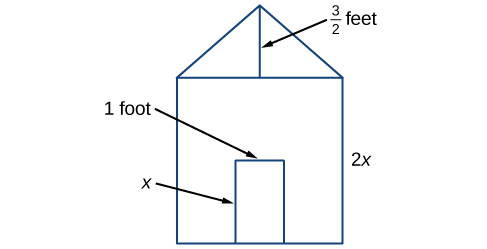## Introduction to Algebraic Operations on Polynomials

Earl is building a doghouse, the front of which is in the shape of a square topped with a triangle. There will be a rectangular door so that Felix, Earl’s dog, can enter and exit the house. In order to save money for treats, Earl wants to buy exactly as much paint as he needs but not any extra. In order to do that, Earl needs to find the area of the front of the doghouse. Using the measurements of the front of the house shown below, we can create a mathematical expression that combines several terms, allowing us to solve this problem and others like it.The front of the doghouse.

First find the area of the square in square feet.

$\begin{array}{l}A\,\,\,=\,\,\,s^{2}\,\,\,\,\,\,\,\,=\,\,\,\left(2x\right)^{2}\,\,\,\,\,\,\,\,=\,\,\,4x^{2}\end{array}$

Then find the area of the triangle in square feet.

$\begin{array}{l}A\,\,\,=\,\,\,\frac{1}{2}bh\\\,\,\,\,\,\,\,\,=\,\,\,\frac{1}{2}\left(2x\right)\left(\frac{3}{2}\right)\\\,\,\,\,\,\,\,\,=\,\,\,\frac{3}{2}x\end{array}$

Next find the area of the rectangular door in square feet.

$\begin{array}{l}A\,\,\,=\,\,\,lw\,\,\,\,\,\,\,\,=\,\,\,x\cdot1\,\,\,\,\,\,\,\,=x\end{array}$

The area of the front of the doghouse can be found by adding the areas of the square and the triangle and then subtracting the area of the rectangle. When we do this, we get

$4{x}^{2}+\frac{3}{2}x-x{\text{ ft}}^{2}=4{x}^{2}+\frac{1}{2}x{\text{ ft}}^{2}$.

In this section, we will examine expressions such as this one, which combine several terms both with and without variables.